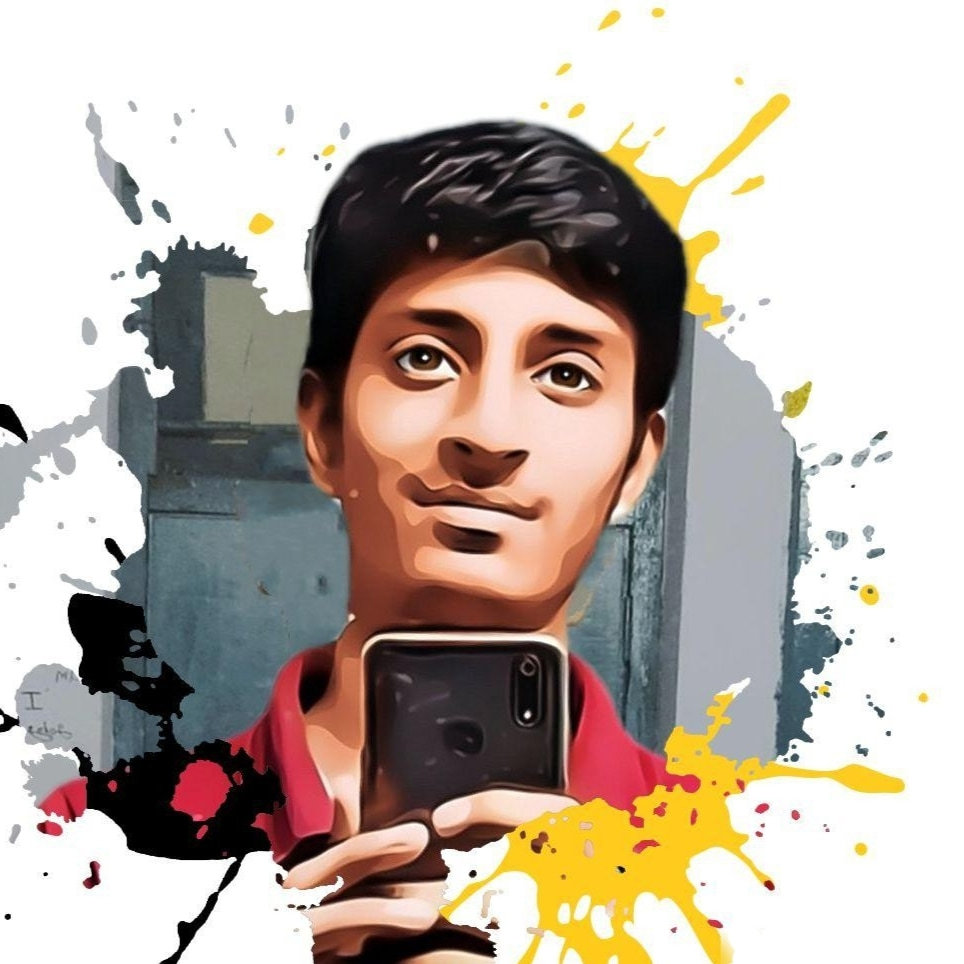# Create a Screen recorder using Python

Python is a widely used general-purpose language. It allows performing a variety of tasks. One of them can be recording a video. It provides a module named pyautogui which can be used for the same. This module along with NumPy and OpenCV provides the way to manipulate and save the images (screenshot in this case)

### Modules needed

• Numpy: To install Numpy type the below command in the terminal.
```pip install numpy
```
• pyautogui: To install pyautogui type the below command in the terminal.
```pip install pyautogui
```
• OpenCV: To install OpenCV type the below command in the terminal.
`pip install opencv-python`

Below is the implementation.

First, import all the required packages.

## Python3

 `# importing the required packages ` `import` `pyautogui ` `import` `cv2 ` `import` `numpy as np`

Now, before recording the screen, we have to create a VideoWriter object. Also, we have to specify the output file name, Video codec, FPS, and video resolution. In video codec, we have to specify a 4-byte code (such as XVID, MJPG, X264, etc.). We’ll be using XVID here.

## Python3

 `# Specify resolution ` `resolution ``=` `(``1920``, ``1080``) ` ` `  `# Specify video codec ` `codec ``=` `cv2.VideoWriter_fourcc(``*``"XVID"``) ` ` `  `# Specify name of Output file ` `filename ``=` `"Recording.avi"` ` `  `# Specify frames rate. We can choose  ` `# any value and experiment with it ` `fps ``=` `60.0` ` `  `# Creating a VideoWriter object ` `out ``=` `cv2.VideoWriter(filename, codec, fps, resolution)`

Optional: To display the recording in real-time, we have to create an Empty window and resize it.

## Python3

 `# Create an Empty window ` `cv2.namedWindow(``"Live"``, cv2.WINDOW_NORMAL) ` ` `  `# Resize this window ` `cv2.resizeWindow(``"Live"``, ``480``, ``270``)`

Now, let’s start recording our screen. We will be running an infinite loop and in each iteration of the loop, we will take a screenshot and write it to the output file with the help of the video writer.

## Python3

 `while` `True``: ` ` `  `    ``# Take screenshot using PyAutoGUI ` `    ``img ``=` `pyautogui.screenshot() ` ` `  `    ``# Convert the screenshot to a numpy array ` `    ``frame ``=` `np.array(img) ` ` `  `    ``# Convert it from BGR(Blue, Green, Red) to ` `    ``# RGB(Red, Green, Blue) ` `    ``frame ``=` `cv2.cvtColor(frame, cv2.COLOR_BGR2RGB) ` ` `  `    ``# Write it to the output file ` `    ``out.write(frame) ` `     `  `    ``# Optional: Display the recording screen ` `    ``cv2.imshow(``'Live'``, frame) ` `     `  `    ``# Stop recording when we press 'q' ` `    ``if` `cv2.waitKey(``1``) ``=``=` `ord``(``'q'``): ` `        ``break`

After everything is done, we will release the writer and destroy all windows opened by OpenCV.

## Python3

 `# Release the Video writer ` `out.release() ` ` `  `# Destroy all windows ` `cv2.destroyAllWindows()`

Full Code:

## Python3

 `# importing the required packages ` `import` `pyautogui ` `import` `cv2 ` `import` `numpy as np ` ` `  `# Specify resolution ` `resolution ``=` `(``1920``, ``1080``) ` ` `  `# Specify video codec ` `codec ``=` `cv2.VideoWriter_fourcc(``*``"XVID"``) ` ` `  `# Specify name of Output file ` `filename ``=` `"Recording.avi"` ` `  `# Specify frames rate. We can choose any  ` `# value and experiment with it ` `fps ``=` `60.0` ` `  ` `  `# Creating a VideoWriter object ` `out ``=` `cv2.VideoWriter(filename, codec, fps, resolution) ` ` `  `# Create an Empty window ` `cv2.namedWindow(``"Live"``, cv2.WINDOW_NORMAL) ` ` `  `# Resize this window ` `cv2.resizeWindow(``"Live"``, ``480``, ``270``) ` ` `  `while` `True``: ` `    ``# Take screenshot using PyAutoGUI ` `    ``img ``=` `pyautogui.screenshot() ` ` `  `    ``# Convert the screenshot to a numpy array ` `    ``frame ``=` `np.array(img) ` ` `  `    ``# Convert it from BGR(Blue, Green, Red) to ` `    ``# RGB(Red, Green, Blue) ` `    ``frame ``=` `cv2.cvtColor(frame, cv2.COLOR_BGR2RGB) ` ` `  `    ``# Write it to the output file ` `    ``out.write(frame) ` `     `  `    ``# Optional: Display the recording screen ` `    ``cv2.imshow(``'Live'``, frame) ` `     `  `    ``# Stop recording when we press 'q' ` `    ``if` `cv2.waitKey(``1``) ``=``=` `ord``(``'q'``): ` `        ``break` ` `  `# Release the Video writer ` `out.release() ` ` `  `# Destroy all windows ` `cv2.destroyAllWindows()`

Output:

Attention geek! Strengthen your foundations with the Python Programming Foundation Course and learn the basics.

To begin with, your interview preparations Enhance your Data Structures concepts with the Python DS Course.

My Personal Notes arrow_drop_up